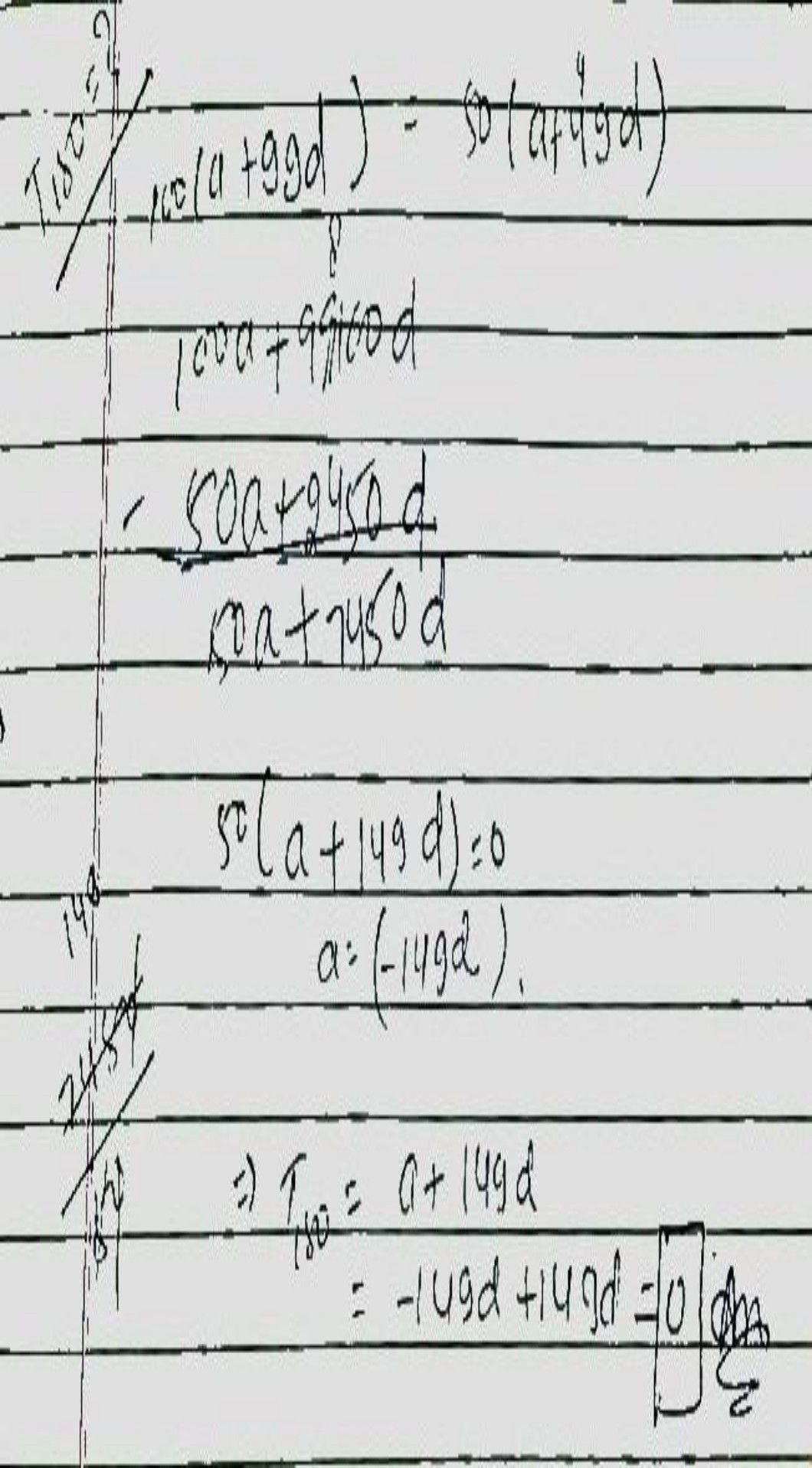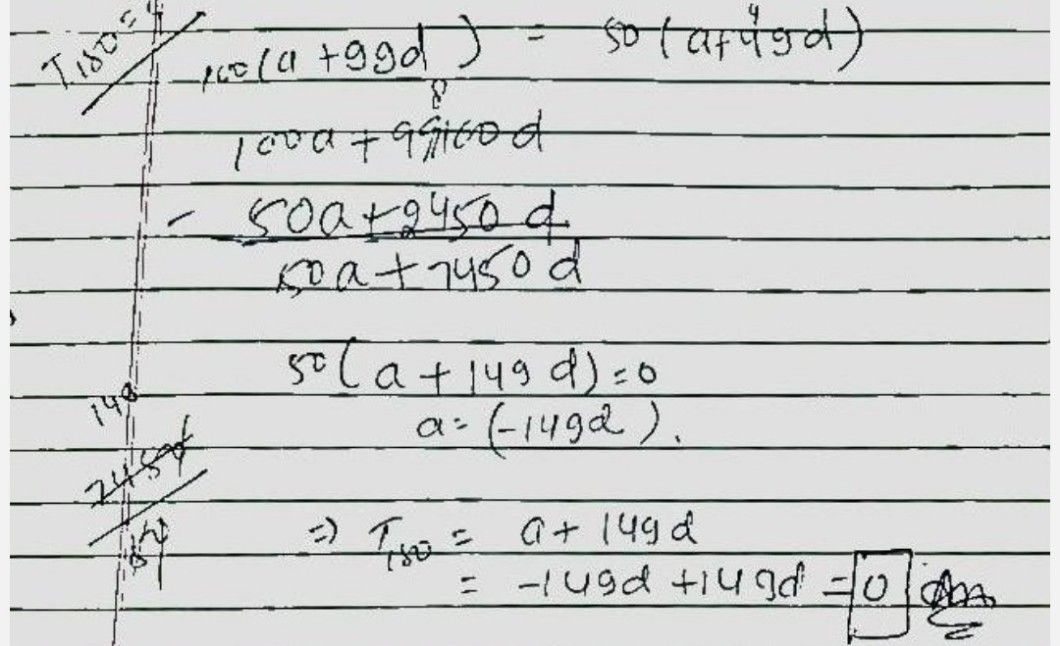Symbol
ProblemIf $100$ times $100tn$ term of an $A$ $A.P$ is equal to $50$ times of its $50tn$ term then $150tn$ term of an $A.P$ is $A$ $150$ $B$ $0$ $C$ $-150$ $D$ $100$
Other
Question content